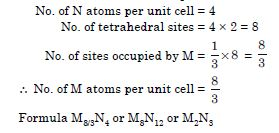# NCERT Solutions for Class 12 Chemistry Chapter 1 - The Solid State

Students of Class 12 can get on with their board exams preparation with NCERT Solutions available at Aasoka, an online learning platform. This platform caters to the need of students. Therefore, Aasoka provides free NCERT Solutions for Class 12 so that students can study efficiently to score good marks in their board exams. The subject matter experts have carefully designed the solutions by including the content as per the latest CBSE syllabus.

“The Solid State” chapter of Class 12 Chemistry provides an explanation of the topics like classification of solids, characteristics of solid state, imperfections in solids, packing in solids, unit cell in two-dimensional and three-dimensional lattices, point defects, crystalline, amorphous, classification of solids based on different binding forces, packing efficiency, voids, and so much more.

##### Question 1:

A compound is formed by two elements X and Y. Atoms of element Y (as anions) make ccp and those of the element X (as cations) occupy all the octahedral voids. What is the formula of the compound?

Suppose number of atoms of Y in ccp = N
No. of octahedral voids = N
No. of atoms of X = N
Formula : XY

##### Question 2:

Atoms of element B form hcp lattice and those of the element A occupy 2/3rd of the tetrahedral voids. What is the formula of the compound formed by these elements A and B?

Atoms B adopt hcp arrangement and there are two tetrahedral sites per atom of B. Since 2/3rd of the tetrahedral sites are occupied by atoms of element A, then for each atom of B, the number of A atoms will be 2 × 2/3 = 4/3.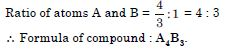##### Question 3:

An element has a body centred cubic (bcc) structure with a cell edge of 288 pm. The density of the element is 7.2 g cm–3. How many atoms are present in 208 g of the element ?

Edge length of the unit cell = 288 pm
= 288 × 10–10 cm
Volume of the unit cell = (288 × 10–10)3 cm3
= 2.39 × 10–23 cm3
Mass of element = 208 g
Density of element = 7.2 g cm–3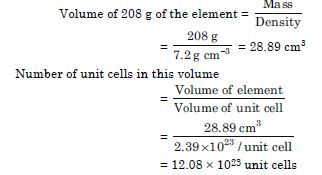Since the structure is bcc, number of atoms present in a unit cell = 2 Total number of atoms in 208 g of the element = 2 × 12.08 × 1023 = 24.16 × 1023 atoms or = 2.416 × 1024 atoms

##### Question 4:

X-ray diffraction studies show that copper crystallizes in an fcc arrangement with cell edge of 3.608 × 10–8 cm. The density of copper has been found to be 8.92 g cm–3. Calculate the atomic (molar) mass of copper.

Edge length of the unit cell = 3.608 × 10–8 cm
Volume of the unit cell = (3.608 × 10–8)3 cm3
Density of the unit cell = 8.92 g cm–3
No. of atoms per unit cell in fcc arrangement,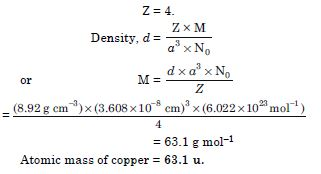##### Question 5:

Silver forms ccp lattice and X-rays studies of its crystals show that the edge length of the unit cell is 408.6 pm. Calculate the density of silver (atomic mass = 107.9 u)

Length of edge, a = 408.6 pm = 408.6 × 10–10 cm
Since the lattice is ccp, the number of atoms per unit cell, Z = 4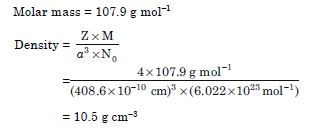##### Question 6:

Why are solids rigid ?

In solids, the particles are closely packed and the empty spaces between the particles are very small. Therefore, solids are incompressible and they maintain their own shape when subjected to outside force. Hence, solids are rigid.

##### Question 7:

Why do solids have a definite volume ?

The intermolecular forces between the particles in the solid state are very strong. Therefore, they are strongly held at fixed positions and particles cannot separate from one another. Hence, solids have a definite volume.

##### Question 8:

Why do solids have a definite volume ?

The intermolecular forces between the particles in the solid state are very strong. Therefore, they are strongly held at fixed positions and particles cannot separate from one another. Hence, solids have a definite volume.

##### Question 9:

Classify the following as amorphous or crystalline solids :

Polyurethane, nephthalene, benzoic acid, teflon, potassium nitrate, cellophane, polyvinyl chloride, fibre glass, copper.

Amorphous solids : Polyurethane, teflon, cellophane, polyvinyl chloride, fibre glass.

Crystalline solids : naphthalene, benzoic acid, potassium nitrate, copper.

##### Question 10:

Refractive index of a solid is observed to have the same value along all directions.

Comment on the nature of this solid. Would it show cleavage property ?

Since refractive index of the solid has same value in all directions i.e., isotropic, it is an amorphous solid. It will not show cleavage property. When cut with a sharp edged tool (knife) it will cut into two pieces with irregular surfaces.

##### Question 11:

Classify the following solids in different categories based on the nature of intermolecular forces operating in them :

Potassium sulphate, tin, benzene, urea, ammonia, water, zinc sulphide, graphite, rubidium, argon, silicon carbide.

Potassium sulphate : ionic solid : tin : metallic solid; benzene : molecular solid : urea : molecular solid; ammonia : molecular solid : water : molecular solid; zinc sulphide : ionic solid : graphite : covalent solid; rubidium

##### Question 12:

Solid A is very hard electrical insulator in solid as well as in molten state and melts at extremely high temperature. What type of solid is it ?

Covalent or network soild like quartz (SiO2), SiC or C (diamond).

##### Question 13:

Ionic solids conduct electricity in molten state but not in solid state. Explain.

In solid state, the ions are present in fixed positions in the crystal lattice and cannot move when electric field is applied. However, when melted, the well ordered arrangement of the ions in the crystal is destroyed and the ions are in a position to move about when an electric current is applied. Hence, ionic solids conduct electricity in molten state.

##### Question 14:

What type of solids are electrical conductors, malleable and ductile ?

Metallic solids.

##### Question 15:

Give the significance of lattice point.

Lattice points represent the positions of the constituent particles (atoms, molecules or ions) in a crystal lattice.

##### Question 16:

Name the parameters that characterize a unit cell.

A unit cell is characterized by the two types of parameters :

1. Dimensions along the three edges represented as a, b and c.
2. Angles between edges $\mathrm{\alpha }$ (between b and c), b (between a and c) and g (between a and b).

Hence a unit cell is represented by six parameters a, b, c, $\mathrm{\alpha }$, $\mathrm{\beta }$, and $\mathrm{\gamma }$.

##### Question 17:

Distinguish between :

1. Hexagonal and monoclinic unit cell
2. Face centred and end centred unit cell
1. Hexagonal unit cell has two edges of equal length (a = b $\ne$ c) while monoclinic unit cell has all
the three edges of unequal length ($\mathrm{\alpha }$ $\ne$ $\mathrm{\beta }$ $\ne$ c).
In hexagonal unit cell two angles are of 90$°$ and one angle of 120$°$ (a = b = 90$°$, g = 120$°$). In monoclinic two angles are of 90$°$ but one is not of 90$°$ ($\mathrm{\alpha }$ = $\mathrm{\gamma }$ = 90$°$, b $\ne$ 90$°$).
2. Face centred unit cell has points at the corners as well as at the centre of each face. It has 4 atoms per unit cell.
End centred unit cell has points at all the corners and at the centre of any two opposite faces. It has 2 atoms per unit cell.

##### Question 18:

Explain how much portion of an atom located at :

(i) corner and (ii) body centre of a cubic unit cell is part of its neighbouring unit cell?

1. 1/8 part of an atom located at corner belongs to neighbouring unit cell
2. No part.
##### Question 19:

What is the two dimensional coordination number of a molecule in square close packed layer?

4

##### Question 20:

A compound forms hexagonal close packed structure. What is the total number of voids in 0.5 mol of it ? How many of these are tetrahedral voids.

An atom in help structure has three voids, one octahedral and two tetrahedral
Number of atoms in 0.5 mol = 0.5 × 6.022 × 1023
= 3.011 × 1023
Total number of voids = 3 × 3.011 × 1023
= 9.033 × 1023
Number of tetrahedral voids = 2 × 3.011 × 1023
= 6.022 × 1023

##### Question 21:

A compound is formed by two elements M and N. The element N forms ccp and M atoms occupy 1/3 of tetrahedral voids. What is the formula of the compound ?

Since N forms ccp arrangement, it will have 4 atoms in a unit cell.

Number of N atoms in unit cell = 4
For each atom, there are two tetrahedral voids so that there are 8 tetrahedral voids per unit cell.

##### Question 22:

Which of the following lattices has the highest packing efficiency (i) simple cubic (ii) body centred cubic and (iii) hexagonal close packed lattice ?

The packing efficiencies are :
Simple cubic = 52.4%
Body centred cubic = 68%
Hexagonal close packed = 74%
\ Hexagonal close packed lattice has highest packing efficiency.

##### Question 23:

An element with molar mass 2.7 × 10–2 kg mol–1 forms a cubic unit cell with edge length 405 pm. If the density is 2.7 × 103 kg m–3. What is the nature of the cubic unit cell ?

Edge length = 405 pm = 405 × 10–12 m
Density of the cell = 2.7 × 103 kgm–3
Molar mass = 2.7 × 10–2 kg mol–1
Z = ?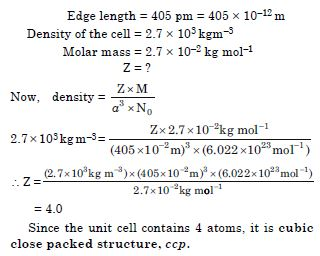##### Question 24:

What type of defect can arise when a solid is heated ? Which physical property is affected by it and in what way ?

Electronic imperfections. These defects affect electrical conductivity. It increases with rise in temperature.

##### Question 25:

What type of stoichiometric defect is shown by:
(i) ZnS (ii) AgBr

1. ZnS : Frenkel defect.
2. AgBr : Frenkel defect as well as Schottky defect.
##### Question 26:

Explain how vacancies are introduced in an ionic solid when a cation of higher valence is added as an impurity in it?

When a cation of higher valence is added to an ionic solid it results into impurity defect and cationic vacancies are introduced to maintain electrical neutrality. For example, when molten NaCl is allowed to crystallise in the presence of SrCl2, some of the Na+ ions are replaced by Sr2+ ions. Each Sr2+ ion replaces two Na+ ions to maintain electrical neutrality. One of the site is occupied by Sr2+ ion and the other site remains vacant. Hence, cationic vacancies are produced equal to the number of Sr2+ ions.

##### Question 27:

Ionic solids which have anionic vacancies due to metal excess defect develop colour. Explain with the help of a suitable example.

When crystals of alkali metal halides such as NaCl are heated in the atmosphere of sodium vapour, the excess of sodium atoms are deposited on the surface of the crystal. The Cl ions diffuse to the surface and combine with sodium atoms to form NaCl. This happens by the loss of electrons by Na atoms to form Na+ ions. The released electrons diffuse into the crystal and occupy anionic sites. As a result, the structure has an excess of sodium. The crystals acquire yellow colour because when visible light falls on the crystals, they absorb energy from the visible region to excite the electrons. Hence, they are coloured. Similarly, excess of Li makes LiCl crystals pink and excess of K makes KCl crystals violet.

##### Question 28:

What type of substances would make better permanent magnets, ferromagnetic or ferrimagnetic ? Justify your answer.

Ferromagnetic substances. When the substance is placed in magnetic field all the domains get oriented in the direction of the magnetic field.

##### Question 29:

Define the term ‘amorphous’. Give a few examples of amorphous solids.

A solid is said to be amorphous if the constituent particles are not arranged in any regular fashion. They may have short range order. For example, glass, plastics, amorphous silica.

##### Question 30:

What makes a glass different from a solid such as quartz ? Under what conditions could quartz be converted into glass ?

Quartz is a crystalline solid in which SiO4 tetrahedral units are arranged in an orderly arrangement. Glass is a supercooled liquid and is an amorphous solid. In which SiO4 tetrahedral units are not arranged in any regular order.

Quartz can be converted into glass by melting the quartz and then cooling it rapidly.

##### Question 31:

Classify each of the following solids as ionic, metallic, molecular, network (covalent) or amorphous.

1. Tetra phosphorus decoxide (P4O10)
2. Graphite
3. Brass
4. Ammonium phosphate (NH4)3PO4
5. SiC
6. Rb
7. I2
8. LiBr
9. P4
10. Si
11. Plastic

The solids are classified as :
Ionic : (NH4)3PO4, LiBr
Metallic : Brass, Rb
Molecular : P4O10, I2, P4, solid CO2
Network (covalent) : Graphite, SiC, Si
Amorphous : Plastics.

##### Question 32:

What is meant by term ‘coordination number’ ?
(b) What is the coordination number of atoms :

1. in a cubic close packed structure
2. in a body centred cubic structure.

(a) Coordination number gives the number of nearest neighbours with which a given atom is in contact. In case of ionic crystals, coordination number of an ion in the crystal is the number of oppositely charged ions surrounding that ion.

(b) (i) 12 (ii) 8

##### Question 33:

How can you determine the atomic mass of an unknown metal if you know its density and the dimension of its unit cell ? Explain.

Consider a unit cell of edge a cm
Volume of unit cell = a3 cm3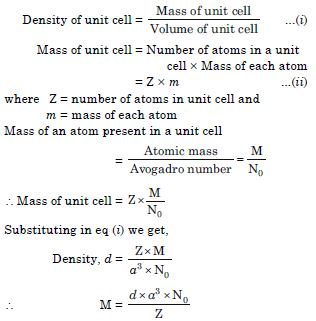Knowing density (d), edge length (a), number of atoms per unit cell (Z) and Avogadro’s number (6.02 × 1023), atomic mass can be calculated.

##### Question 34:

‘Stability of a crystal is reflected in the magnitude of its melting points’. Comment.

Collect melting points of solid water, ethyl alcohol, diethyl ether and methane from a data book. What can you say about the intermolecular forces between these molecules ?

The melting point of a crystal depends upon the magnitude of forces holding the constituent particles together, which determine the stability. Higher the melting point, greater are the forces holding the constituent particles together and hence greater is the stability. For example, ionic crystals such as NaCl, KNO3, etc. have very high melting points and are stable. On the other hand, molecular solids such as nephthalene, iodine, etc. are less stable because they have low values of melting points.

The melting points of some compounds are :

Water = 273 K, ethyl alcohol = 155.8 K, diethyl ether = 156.8 K and methane = 90.5 K.

The intermolecular forces in water and ethyl alcohol are mainly hydrogen bonding. The higher melting point of water than ethyl alcohol indicates that the hydrogen bonding in water is stronger than in ethyl alcohol. Diethyl ether is a polar molecule and, therefore, the intermolecular forces in diethyl ether are dipole-dipole interactions. On the other hand, methane is a non-polar molecule and the only forces present in them are the weak van der Waals forces (London dispersion forces). These are weaker than dipole-dipole interactions and hence methane has very low melting point than diethyl ether.

##### Question 35:

How will you distinguish between the following pair of terms :

1. Hexagonal close packing and cubic close packing
2. Crystal lattice and unit cell.
3. Tetrahedral void and octahedral void.
1. In hexagonal close packing (hcp) the close packing is ABAB.... type whereas in cubic close packing (ccp), the close packing is ABCABC.... type.
2. The three dimensional arrangement of constituent particles of a substance (atoms, ions or molecules) is called crystal lattice.
The smallest repeating pattern in a crystal lattice which when repeated in three dimensions gives the crystal is called unit cell.
3. A void surrounded by four spheres is called a tetrahedral void while a void surrounded by six spheres is called an octahedral void.
##### Question 36:

How many lattice points are there in one unit cell of each of the following lattice ?

(a) face centred cubic (b) face centred tetragonal (c) body centred.

(a) In face centred cubic arrangement, number of lattice points are :
8 (at corners) + 6 (at face centres)
Lattice points per unit cell =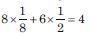(b) In face centred tetragonal, number of lattice points are:
= 8 (at corners) + 6 (at face centres)
Lattice points per unit cell =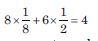(c) In body centred cubic arrangement number of lattice points are :
= 8 (at corners) + 1 (at body center)
Lattice points per unit cell =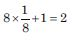##### Question 37:

Explain :

1. The basis of similarities and differences between metallic and ionic crystals.
2. Ionic solids are hard and brittle.

(a) Basis of similarities

1. Both ionic and metallic crystals have electrostatic forces of attraction. In ionic crystals, these are between the oppositely charged ions while in metals, these are among the valence electrons and the kernels. That is why both ionic and metallic crystals have high melting points.
2. In both cases the bond is non-directional.

Basis of differences

1. In ionic crystals, the ions are not free to move and therefore, they do not conduct electricity in the solid state. They conduct electricity in the molten state or in their aqueous solution. However, in metals, the valence electrons are free to move and hance they conduct electricity in the solid state.
2. Ionic bond in ionic crystals is strong due to electrostatic forces of attraction. However, metallic bond may be weak or strong depending upon the number of valence electrons and the size of the kernels.

Ionic crystals are hard because there are strong electrostatic forces of attraction among the oppositely charged ions. They are brittle because the ionic bond is non-directional.

##### Question 38:

Calculate the efficiency of packing in case of a metal crystal for (a) face centred cubic (b) body centred cubic (c) simple cubic (with the assumptions that atoms are touching each other).

(a) Packing efficiency for face centred cubic
Suppose the edge length of the unit cell = a
and radius of the sphere = r
As is clear from the figure (a) that there are 8 spheres at the corners and six spheres at the faces.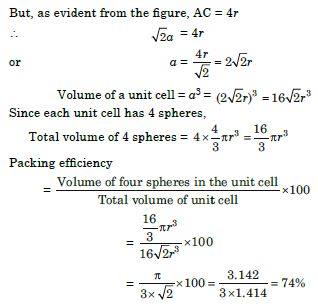(b) Body centred cubic (bcc) arrangement Suppose the edge length = a and radius of each sphere = r As is clear from figure (a) that there are 8 spheres at the corners and one in the body of the unit cell.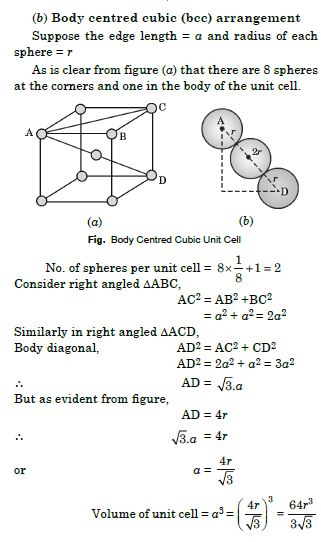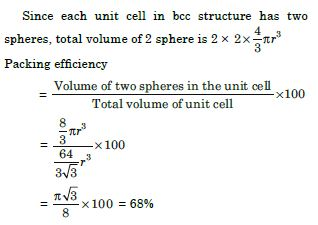(c) In a simple cubic unit cell
Suppose the edge length of the unit cell = a and radius of the sphere = r
Since the spheres are touching each other, a = 2 r
Now, there are eight spheres at the corners of the cube.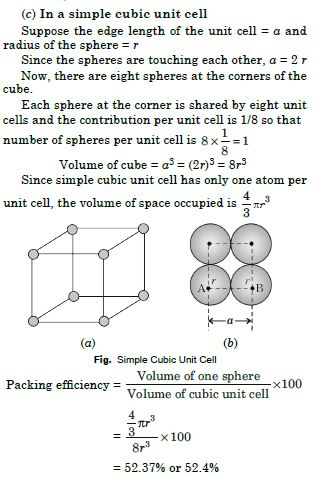##### Question 39:

Silver crystallizes in fcc lattice. If edge length of the cell is 4.077 × 10–8 cm and density is 10.5 g cm–3. Calculate the atomic mass of silver.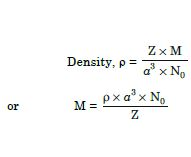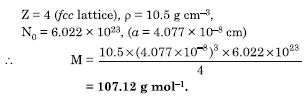##### Question 40:

A cubic solid is made of two elements P and Q. Atoms of Q are at the corners of the cube and P at the body centre. What is the formula of the compound ? What are the coordination numbers of P and Q ?

As atoms Q are present at the 8 corners of the cube, therefore, number of atoms of Q in the unit cell =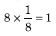As atoms P are present at the body centre, therefore, number of atoms P in the unit cell = 1
\ Formula of the compound = PQ
Coordination number of each P and Q = 8

##### Question 41:

Niobium crystallizes in body centered cubic structure. If density is 8.55 g cm–3, calculate atomic radius of niobium using its atomic mass 93 u.

Density = 8.55 g cm–3 Let length of the edge = a cm Number of atoms per unit cell, Z = 2 (bcc) Atomic mass, M = 93 g mol–1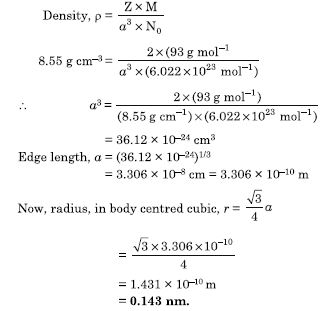##### Question 42:

If the radius of the octahedral void is r and radius of the atoms in close packing is R. Derive relation between r and R.

A sphere of radius r filling in an octahedral void of spheres of radius R is shown in figure.
If the length of the unit cell is a cm, then In right angled DABC, AB = BC = a cm
The diagonal AC is :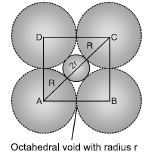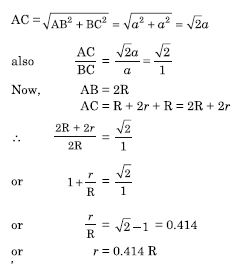Thus, for an atom to occupy an octahedral void, its radius must be 0.414 times the radius of the sphere.

##### Question 43:

Copper crystallizes into a fcc latice with edge length 3.61 × 10–8 cm. Show that the calculated density is in agreement with its measured value of 8.29 g cm–3.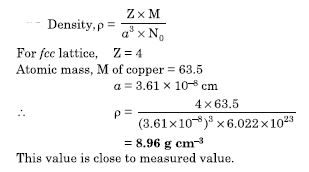##### Question 44:

Analysis shows that nickel oxide has formula Ni0.98O1.00. What fractions of nickel exist as Ni2+ and Ni3+ ions ?

Ni0.98O1.00.
Let Ni2+ be x so that Ni3+ will be 0.98 – x. Total charge on the compound must be zero so that
+ 2x + 3 (0.98 – x) – 2 = 0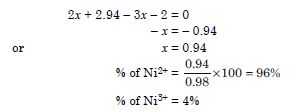##### Question 45:

What is a semiconductor ? Describe the two main types of semiconductors and contrast their conduction mechanism.

The substances whose conductance lies between that of conductors (metals) and insulators are called semiconductors. They have conductivity values ranging from 10–6 to 104 W–1m–1. Two main types of semiconductors are n-type and p-type.

1. n-type semiconductors : These are the semiconductors in which the current is carried by the electrons in the normal way. For example, silicon and germanium belong to group 14 of the periodic table and have four valence electrons each. In their crystals, each atom forms four covalent bonds with its neighbours. When it is doped with an element of group 15 such as P or As, which contains five valence electrons, they occupy some of the lattice sites in silicon or germanium crystal. Four of the five valence electrons are used in the formation of four covalent bonds with the four neighbouring Si atoms and the fifth electron is extra and becomes delocalised. This delocalised electron will be able to conduct and such type of conduction is called n-type conduction. Hence, silicon or germanium doped with extra electron of impurity are called n-type semiconductors.
2. p-type semiconductors : These are the semiconductors in which current is carried by the movement of positive holes. For example, when Ge or Si are doped with electron deficient atoms such as Ga or In (of Group 13) containing three valence electrons, the atoms of Ga or In will replace Ge atoms. Each In or Ga atom will use its three electrons for forming three covalent bonds with neighbouring Ge atoms and the place for fourth bond will remain missing and is called electron vacancy or hole. Such holes can move through the crystals like a positive charge giving rise to conductivity. This type of conduction is called p-type conduction. Hence, Si or Ge doped with electron deficient atoms as impurity are called p-type semiconductors.
##### Question 46:

Non-stoichiometric cuprous oxide, Cu2O can be prepared in laboratory. In this oxide, copper to oxygen ratio is slightly less than 2 : 1. Can you account for the fact that this substance is a p-type semiconductor ?

Since the ratio of Cu : O in Cu2O is less than 2 : 1, therefore, Cu2O is non-stoichiometric crystal. This means that some Cu+ ions have been replaced by Cu2+ ions. To maintain electrical neutrality, every two Cu+ ions will be replaced by one Cu2+ ion thereby creating a hole. Since the conduction will be due to the presence of these positive holes, it is a p-type semiconductor.

##### Question 47:

Ferric oxide crystallizes in a hexagonal close packed array of oxide ions with two out of every three octahedral holes occupied by ferric ions. Derive the formula of the ferric oxide.

In a close packed arrangement, there is one octahedral site corresponding to each atom constituting the lattice.
No. of oxide ions per unit cell in hcp arrangement = 6
No. of octahedral holes = 6
No. of ferric ions =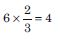##### Question 48:

Classify each of the following as being either a p-type or a n-type semiconductor:
(i) Ge doped with In (ii) B doped with Si

1. Ge belongs to group 14 and In belongs to group 13. Therefore, an electron deficient hole is created and it is a p-type semiconductor.
2. B belongs to group 13 and Si belongs to group 14. Therefore, there will be a free electron and it is a n-type semiconductor.
##### Question 49:

Gold (atomic radius = 0.144 nm) crystallizes in a face centered unit cell. What is the length of a side of the cell?

For face centred unit cell, radius of atom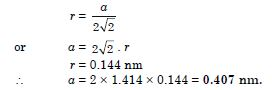##### Question 50:

In terms of band theory, what is the difference (i) between a conductor and an insulator (ii) between a conductor and a semiconductor?

1. The energy gap between the valence band and the conduction band in an insulator is very large whereas in a conductor the energy gap is either very small or there is overlapping between valence band and conduction band.
2. In a conductor, the energy gap between valence band and conductance band is very small or there is overlapping between valence band and conduction band. But in a semiconductor, there is always a small energy gap between them.
##### Question 51:

Explain the following terms with suitable examples :

(i) Schottky defect (ii) Frenkel defect (iii) Interstitials (iv) F-centres

1. Schottky defect : This defect arises if some of the atoms or ions are missing from their normal lattice sites. The lattice sites which are unoccupied are called lattice vacancies or holes. Since the crystal is to remain electrically neutral, equal number of cations and anions are missing. The existence of two holes, one due to a missing cation and the other due to a missing anion, is shown in Fig. 1. Schottky defect is more common in strongly ionic compounds having a high coordination number. For example, NaCl and CsCl ionic solids have Schottky defects. Because of the presence of vacancies in crystals, its density in markedly lowered.
2. Frenkel defect : This defect arises when an ion is missing from its own position and occupies an interstitial site. The existence of one hole due to a missing cation from its position and occupying an interstitial position is shown in Fig. 2. In this case also the crystal remains electrically neutral. Frenkel defects generally occur in compounds in which anions are much larger than the cations and the coordination number is low. These defects can be found in silver halides because of the small size of the Ag+ ion.
##### Question 52:

Aluminium crystallizes in a cubic close packed structure. Its metallic radius is 125 pm.

1. What is the length of the side of the unit cell ?
2. How many unit cells are there in 1.00 cm3 of aluminium ?

(a) For a cubic close packed structure, length of the side of unit cell is related to radius.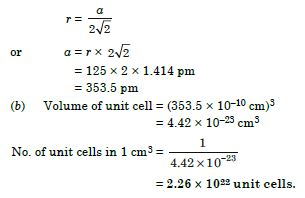##### Question 53:

If NaCl is doped with 10–3 mol % of SrCl2. What is the concentration of cation vacancies?

One cation of Sr2+ would create one cation vacancy in NaCl. Therefore, the number of cation vacancies created in the lattice of NaCl is equal to the number of divalent Sr2+ ions added. \ Concentration of cation vacancy on being doped with 10–3 mol % SrCl2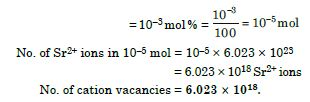##### Question 54:

Explain the following with suitable examples :

1. Ferromagnetism
2. Paramagnetism
3. Ferrimagnetism
4. Anti-ferromagnetism
5. 12–16 and 13–15 group compounds.
1. Ferromagnetism : When there is spontaneous alignment of magnetic moments of domains in the same direction, we get ferromagnetism. These have strong magnetic effects and ordering of domains persists even when magnetic field is removed and ferromagnetic substance becomes permanent magnet.
2. Paramagnetism : The substances which have permanent magnetic dipoles and are attracted by the magnetic field are called paramagnetic substances. This property of attraction by the magnetic field is known as paramagnetism. The paramagnetic substance are atoms, molecules or ions having unpaired electrons. For example, O2, Cu2+, Fe2+, etc. They lose their magnetism in the absence of magnetic field.
3. Ferrimagnetism : When the magnetic moments of the domains are aligned in parallel or anti-parallel directions in unequal numbers resulting in net magnetic moment, we get ferrimagnetism.
4. Anti-ferromagnetism : It arises when the alignment of magnetic moments is in a compensatory way so that the resultant magnetic moment is zero (e.g., MnO).
5. The solid binary compounds prepared by combining elements of group 12 and 16 are called 12 – 16 compounds. For example CdS, ZnS, etc. The compounds prepared by combining elements of group 13 and 15 are called 13–15 compounds. For example, AlP, GaAs, etc.

These compounds are used as semiconductors.

##### Question 55:

Why are liquids and gases categorised as fluids?

The liquids and gases have a property to flow i.e. the molecules of liquids and gases can easily move past and tumble over one another freely. Because of their tendency to flow, these have been categorised as fluids.

##### Question 56:

Why are solids incompressible?

The internuclear distances between the constituent particles (atoms, molecules or ions) in solids are very less. On bringing them further closer, there will be large repulsive forces between electron clouds of these particles. Therefore, solids cannot be compressed.

##### Question 57:

Inspite of long range order in the arrangement of particles why are the crystals usually not perfect?

During the crystallisation process, some deviations from the ideal ordered arrangement may occur. As a result, crystals are usually not perfect.

##### Question 58:

Why does table salt, NaCl, some times appear yellow in colour?

The yellow colour of sodium chloride crystals is due to metal excess defect. In this defect, the unpaired electrons get trapped in anion vacancies. These sites are called F-centres. The yellow colour results by excitation of these electrons when they absorb energy from the visible light falling on the crystals.

##### Question 59:

Why is FeO(s) not formed in stoichiome-tric composition?

In the crystals of FeO, some of the Fe2+ cations are replaced by Fe3+ ions. To balance the charge, three Fe2+ ions are replaced by two Fe3+ ions to make up for the loss of positive charge. As a result, there would be less amount of metal as compared to stoichiometric proportion.

##### Question 60:

Why does white ZnO (s) becomes yellow upon heating?

When ZnO is heated it loses oxygen as :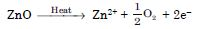The Zn2+ ions are entrapped in the interstitial sites and electrons are entrapped in the neighbouring interstitial sites to maintain electrical neutrality. This results in metal excess defect and F-centres are created. Due to the presence of electrons in the interstitial voids, the colour is yellow.

##### Question 61:

Why does the electrical conductivity of semiconductors increase with rise in temperature?

According to band model, the gap between conduction band and valence band is small in semiconductors.

Therefore, electrons from the valence band can jump to the conduction band on increasing temperature. Thus, they become more conducting as the temperature increases.

##### Question 62:

Explain why does conductivity of germanium crystals increase on doping with gallium.

On doping germanium with gallium, some of the positions of lattice of germanium are occupied by gallium. Gallium atom has only three valence electrons. Therefore, fourth valency of nearby germanium atom is not satisfied and this site remains vacant. This place is deficient of electrons and is called electron hole or electron vacancy. Electron from neighbouring atom moves to fill the gap, thereby creating a hole in its original position. Under the influence of electric field, electrons move towards positively charged plates through these holes and conduct electricity. The holes appear to move towards negatively charged plates. The movement of electrons (or electron holes) results in increase in conductivity of germanium.

##### Question 63:

In a compound, nitrogen atoms (N) make cubic close packed lattice and metal atoms (M) occupy one-third of the tetrahedral voids present. Determine the formula of the compound formed by M and N?